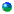Course Description

Introduction to statistical consulting modified

presentation of David Borgerding
(fair use for teaching)

How to load packages in R?

R-Commands for smoothing

Homework2

Regression tree analysis using S-plus.

Commands for regression tree in R (
Note that alpha numeric
variables are coded as a, b, c, d, .... in the alphabetic order, e.g. in the variable Country
a = Germany, b = Japan, c = Japan/USA, d = Korea, e = Mexico, f  = Sweden, g = USA.)
Data for doing regression tree homework
Regression Tree.

Homework3 Regression tree and SAS graphics

Reading for case study: fish corresponding Case01.xls

Homework4 Fisher's exact test and test for independence

Homework5 Test for trend and meta analysis

Homework6 McNemar's test and Kappa.

Homework7 goodness_of_fit test and longitudinal data

Homework8 subgroup analysis and correlation

Homework9 simple regression analysis

Homework10 parametric versus nonparametric analysis

Homework11 Logistic regression

Homework12 Factor Analysis

Final exam Due on or before Friday May 8, 6 pm, 2009

Data2LOESS smoothing exampleSome useful websites for consultingHow to access SAS on afsTrellis graphics through RTrellis graphics through S-plusBasic splus commandsBird data splus commandsDataFisher's exact test from Handbook of Parametric and Nonparametric Statistical Procedures by David J.Sheskin P1 P2 P3 P4 P5 P6SAS Example 1SAS Example 2SAS Example 3SAS logistic regression and odds ratioLatin SquareChi-square, Goodness of fit, independence, homogeneity
When is the test statistics approximately Chi-squaredMcNemar's TestNotes on Kappa from Medical Statistics by Machin,
Campbell,Waltersindependent samples t & non parametric tests SASTest of Proportion(s)Case study #1Sample Survey
pg2Some references